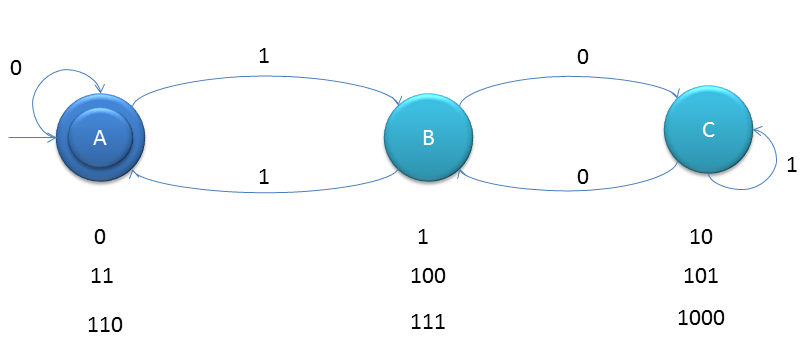# DFA String Examples

Construct a minimal DFA, which accepts set of all strings over {0, 1}, which when interpreted as binary number is divisible by ‘3’.
Means 110 in binary is equivalent to 6 in decimal and 6 is divisible by 3.

So if you think in the way of considering remainders if you divide by 3 that is {0, 1, 2}As you can see that binary number which is divisible by 2 is appearing on left state. Short Trick to create such DFAs
Create Transition Table as below
State01
q0q0q1
q1q2q0
q2q1q2
Table creation rules
1. first write the input alphabets, example 0, 1
2. there will n states for given number which is divisor.
3. start writing states, as for n=3: q0 under 0, q1 under 1
4. q2 under 0 and q0 under 1
5. q1under 0 and q2 under 1
If the input alphabets are 0, 1, 2 then table will expand accordingly with same rules above. Example
For ternary pattern and n=4, table will be as follows ;
State012
q0q0q1q2
q1q3q0q1
q2q2q3q0
q3q1q2q3